# Creating a confusion matrix with cvms

#### 2021-11-14

Abstract

In this vignette, we learn how to create and plot a confusion matrix from a set of classification predictions. The functions of interest are evaluate() and plot_confusion_matrix().

Contact the author at

# Introduction

When inspecting a classification model’s performance, a confusion matrix tells you the distribution of the predictions and targets.

If we have two classes (0, 1), we have these 4 possible combinations of predictions and targets:

Target Prediction Called*
0 0 True Negative
0 1 False Positive
1 0 False Negative
1 1 True Positive

* Given that 1 is the positive class.

For each combination, we can count how many times the model made that prediction for an observation with that target. This is often more useful than the various metrics, as it reveals any class imbalances and tells us which classes the model tend to confuse.

An accuracy score of 90% may, for instance, seem very high. Without the context though, this is impossible to judge. It may be, that the test set is so highly imbalanced that simply predicting the majority class yields such an accuracy. When looking at the confusion matrix, we discover many of such problems and gain a much better intuition about our model’s performance.

In this vignette, we will learn three approaches to making and plotting a confusion matrix. First, we will manually create it with the table() function. Then, we will use the evaluate() function from cvms. This is our recommended approach in most use cases. Finally, we will use the confusion_matrix() function from cvms. All approaches result in a data frame with the counts for each combination. We will plot these with plot_confusion_matrix() and make a few tweaks to the plot.

Let’s begin!

## Attach packages and set seed for reproducibility

library(cvms)
library(tibble)   # tibble()

set.seed(1)

## Binomial data

We will start with a binary classification example. For this, we create a data frame with targets and predictions:

d_binomial <- tibble("target" = rbinom(100, 1, 0.7),
"prediction" = rbinom(100, 1, 0.6))

d_binomial
#> # A tibble: 100 × 2
#>    target prediction
#>     <int>      <int>
#>  1      1          0
#>  2      1          1
#>  3      1          1
#>  4      0          0
#>  5      1          0
#>  6      0          1
#>  7      0          1
#>  8      1          1
#>  9      1          0
#> 10      1          1
#> # … with 90 more rows

# Manually creating a two-class confusion matrix

Before taking the recommended approach, let’s first create the confusion matrix manually. Then, we will simplify the process with first evaluate() and then confusion_matrix(). In most cases, we recommend that you use evaluate().

Given the simplicity of our data frame, we can quickly get a confusion matrix table with table():

basic_table <- table(d_binomial)
basic_table
#>       prediction
#> target  0  1
#>      0 15 17
#>      1 25 43

In order to plot it with ggplot2, we convert it to a data frame with parameters::model_parameters():

cfm <- as_tibble(basic_table)
cfm
#> # A tibble: 4 × 3
#>   target prediction     n
#>   <chr>  <chr>      <int>
#> 1 0      0             15
#> 2 1      0             25
#> 3 0      1             17
#> 4 1      1             43

We can now plot it with plot_confusion_matrix():

plot_confusion_matrix(cfm,
target_col = "target",
prediction_col = "prediction",
counts_col = "n")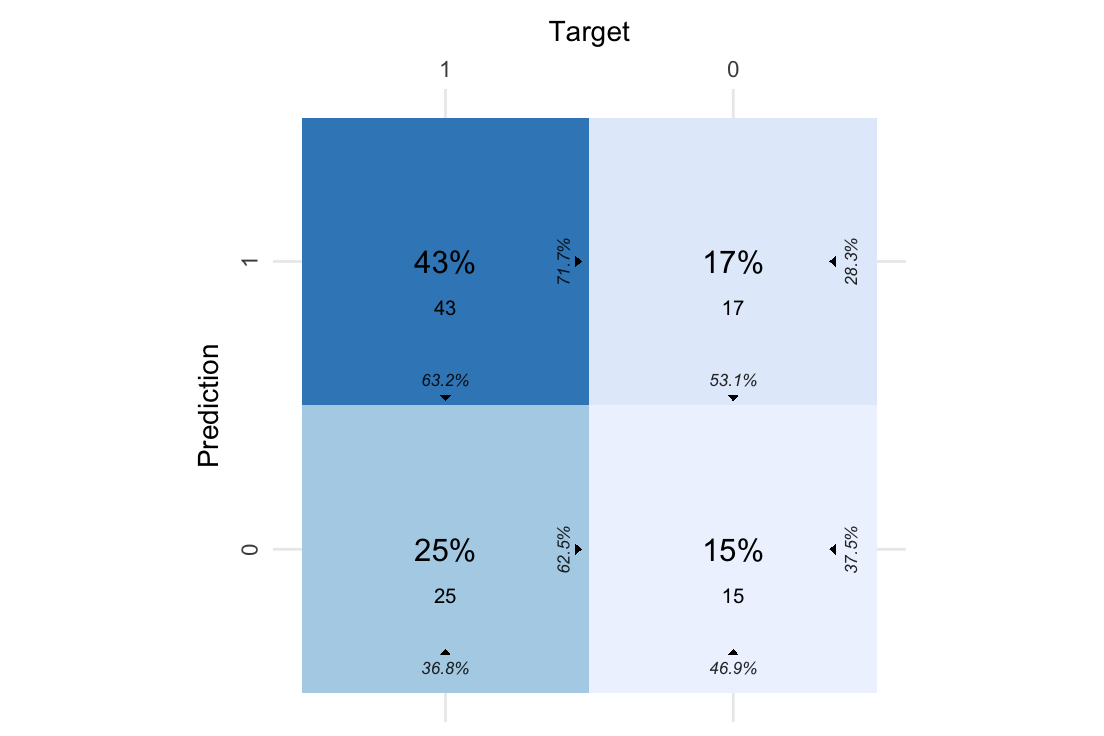In the middle of each tile, we have the normalized count (overall percentage) and, beneath it, the count.

At the bottom, we have the column percentage. Of all the observations where Target is 1, 63.2% of them were predicted to be 1 and 36.8% 0.

At the right side of each tile, we have the row percentage. Of all the observations where Prediction is 1, 71.7% of them were actually 1, while 28.3% were 0.

Note that the color intensity is based on the counts.

Now, let’s use the evaluate() function to evaluate the predictions and get the confusion matrix tibble:

# Creating a confusion matrix with evaluate()

eval <- evaluate(d_binomial,
target_col = "target",
prediction_cols = "prediction",
type = "binomial")

eval
#> # A tibble: 1 × 19
#>   Balanced Accuracy Accuracy    F1 Sensitivity Specificity Pos Pred Value
#>                 <dbl>    <dbl> <dbl>       <dbl>       <dbl>            <dbl>
#> 1               0.551     0.58 0.672       0.632       0.469            0.717
#> # … with 13 more variables: Neg Pred Value <dbl>, AUC <dbl>, Lower CI <dbl>,
#> #   Upper CI <dbl>, Kappa <dbl>, MCC <dbl>, Detection Rate <dbl>,
#> #   Detection Prevalence <dbl>, Prevalence <dbl>, Predictions <list>,
#> #   ROC <named list>, Confusion Matrix <list>, Process <list>

The output contains the confusion matrix tibble:

conf_mat <- eval$Confusion Matrix[] conf_mat #> # A tibble: 4 × 5 #> Prediction Target Pos_0 Pos_1 N #> <chr> <chr> <chr> <chr> <int> #> 1 0 0 TP TN 15 #> 2 1 0 FN FP 17 #> 3 0 1 FP FN 25 #> 4 1 1 TN TP 43 Compared to the manually created version, we have two extra columns, Pos_0 and Pos_1. These describe whether the row is the True Positive, True Negative, False Positive, or False Negative, depending on which class (0 or 1) is the positive class. Once again, we can plot it with plot_confusion_matrix(): plot_confusion_matrix(conf_mat)# Multiclass confusion matrix with confusion_matrix() A third approach is to use the confusion_matrix() function. It is a lightweight alternative to evaluate() with fewer features. As a matter of fact, evaluate() uses it internally! Let’s try it on a multiclass classification task. Create a data frame with targets and predictions: d_multi <- tibble("target" = floor(runif(100) * 3), "prediction" = floor(runif(100) * 3)) d_multi #> # A tibble: 100 × 2 #> target prediction #> <dbl> <dbl> #> 1 0 2 #> 2 0 0 #> 3 1 1 #> 4 0 1 #> 5 0 1 #> 6 1 2 #> 7 1 0 #> 8 0 2 #> 9 0 0 #> 10 2 1 #> # … with 90 more rows Whereas evaluate() takes a data frame as input, confusion_matrix() takes a vector of targets and a vector of predictions: conf_mat <- confusion_matrix(targets = d_multi$target,
predictions = d_multi$prediction) conf_mat #> # A tibble: 1 × 15 #> Confusion Matrix Table Class Level Res… Overall Accura… Balanced Accur… #> <list> <list> <list> <dbl> <dbl> #> 1 <tibble [9 × 3]> <table… <tibble [3 × 14]> 0.34 0.502 #> # … with 10 more variables: F1 <dbl>, Sensitivity <dbl>, Specificity <dbl>, #> # Pos Pred Value <dbl>, Neg Pred Value <dbl>, Kappa <dbl>, MCC <dbl>, #> # Detection Rate <dbl>, Detection Prevalence <dbl>, Prevalence <dbl> The output includes the confusion matrix tibble and related metrics. Let’s plot the multiclass confusion matrix: plot_confusion_matrix(conf_mat$Confusion Matrix[])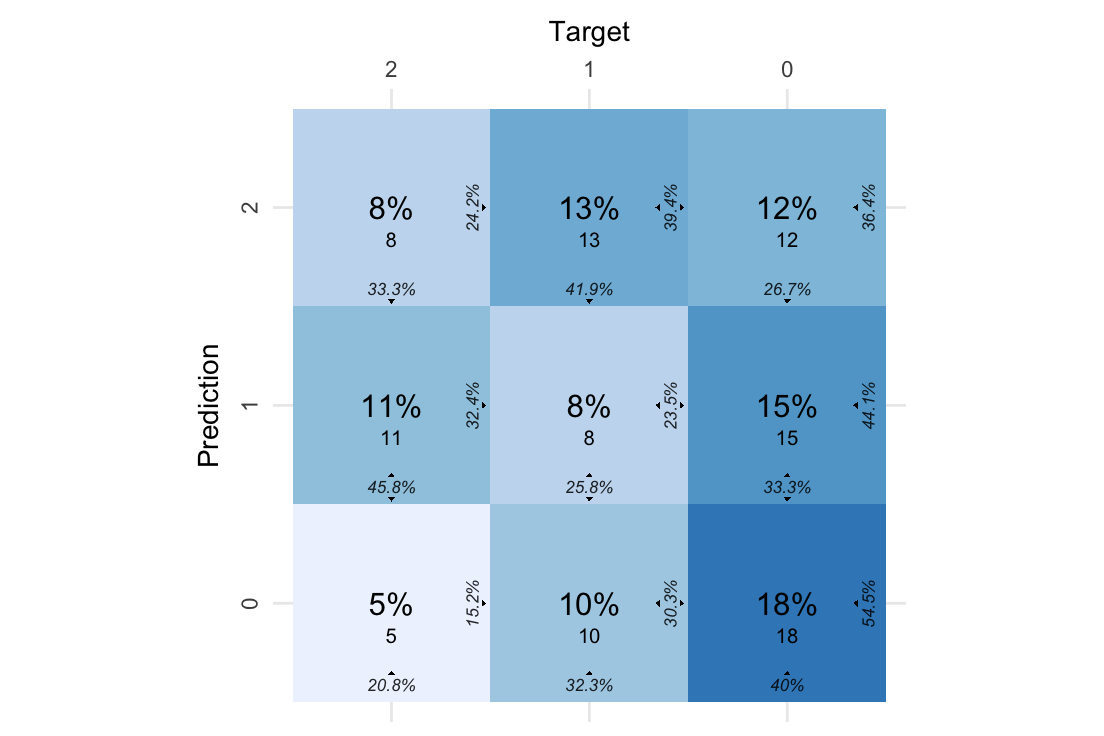If we are interested in the overall distribution of predictions and targets, we can add a column to the right side of the plot with the row sums and a row at the bottom with the column sums. We refer to these as the sum tiles.

plot_confusion_matrix(conf_mat$Confusion Matrix[], add_sums = TRUE)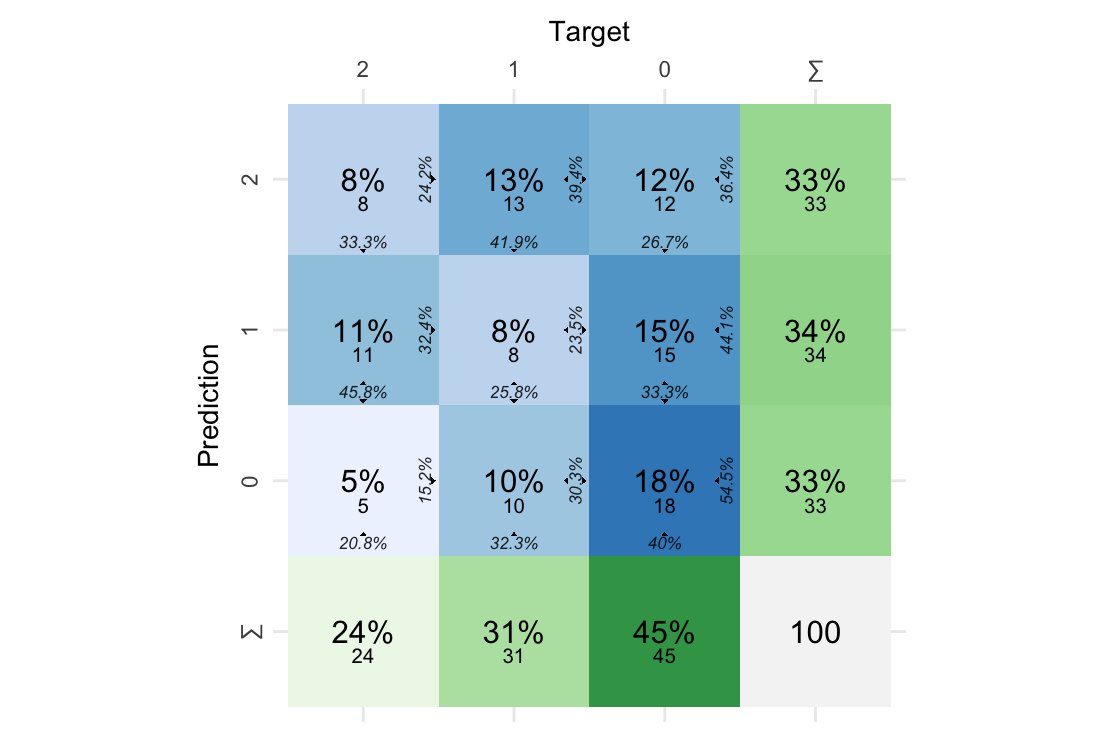The tile in the corner contains the total count of data points. # Tweaking plot_confusion_matrix() Let’s explore how we can tweak the plot. While the defaults of plot_confusion_matrix() should (hopefully) be useful in most cases, it is very flexible. For instance, you may prefer to have the “Target” label at the bottom of the plot: plot_confusion_matrix(conf_mat$Confusion Matrix[],
place_x_axis_above = FALSE)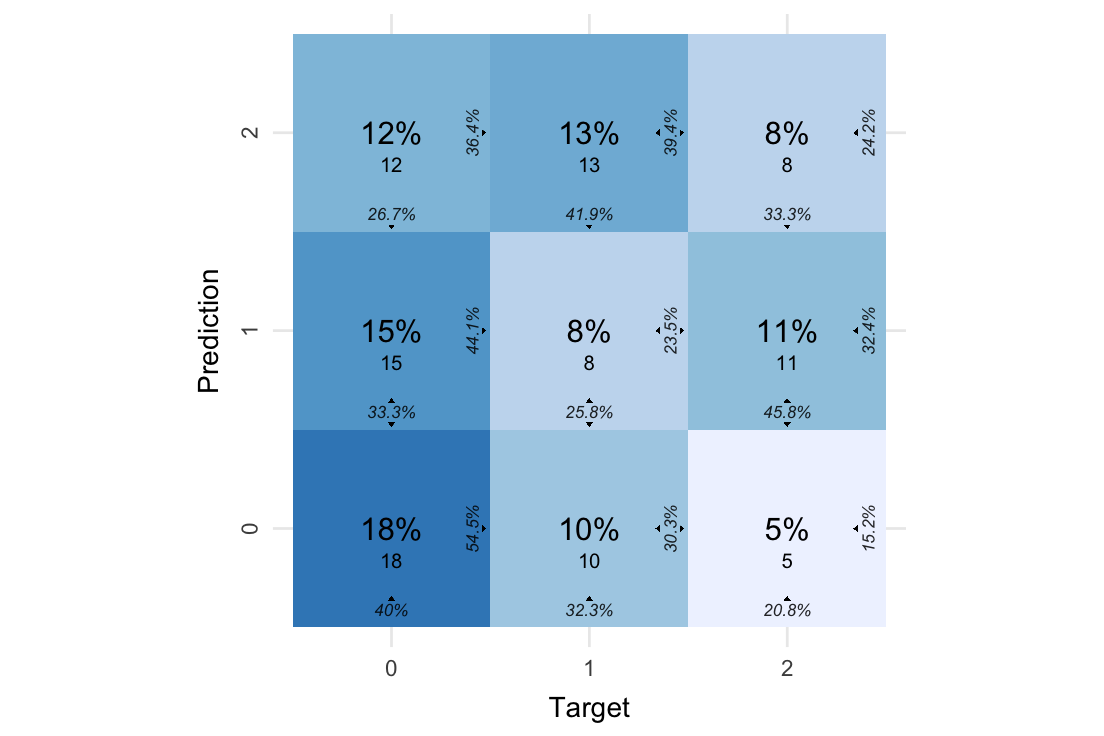If we only want the counts in the middle of the tiles, we can disable the normalized counts (overall percentages):

plot_confusion_matrix(conf_mat$Confusion Matrix[], add_normalized = FALSE)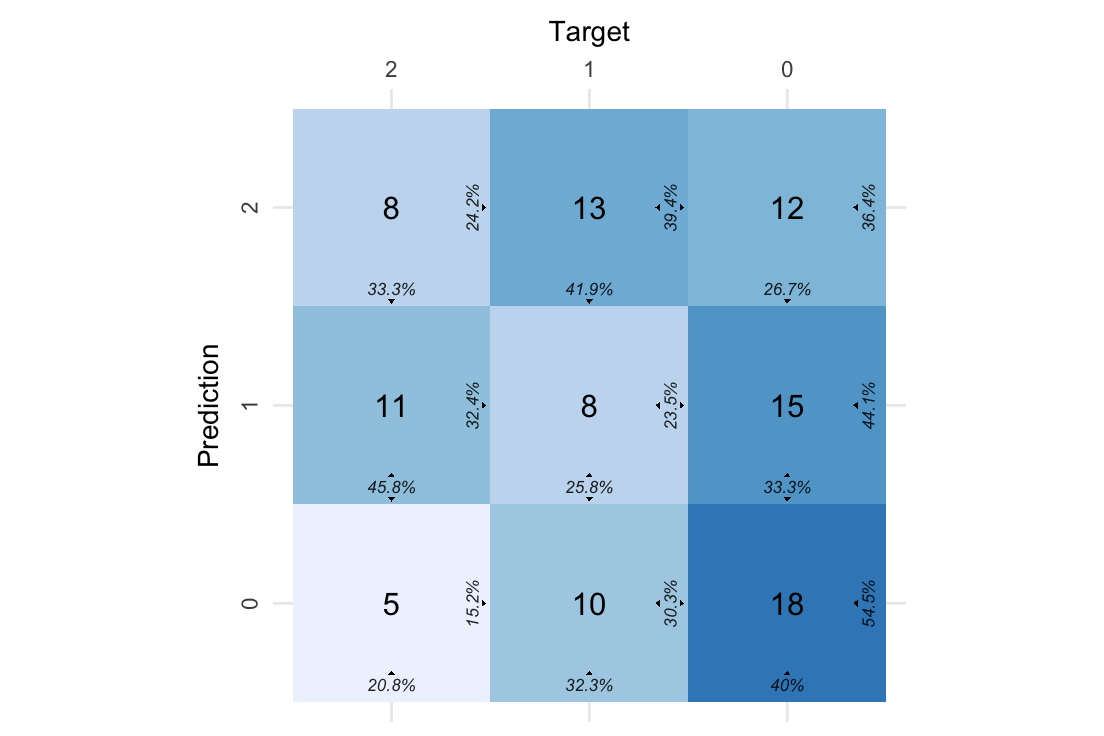We can choose one of the other available color palettes. You can find the available sequential palettes at ?scale_fill_distiller. plot_confusion_matrix(conf_mat$Confusion Matrix[],
palette = "Oranges")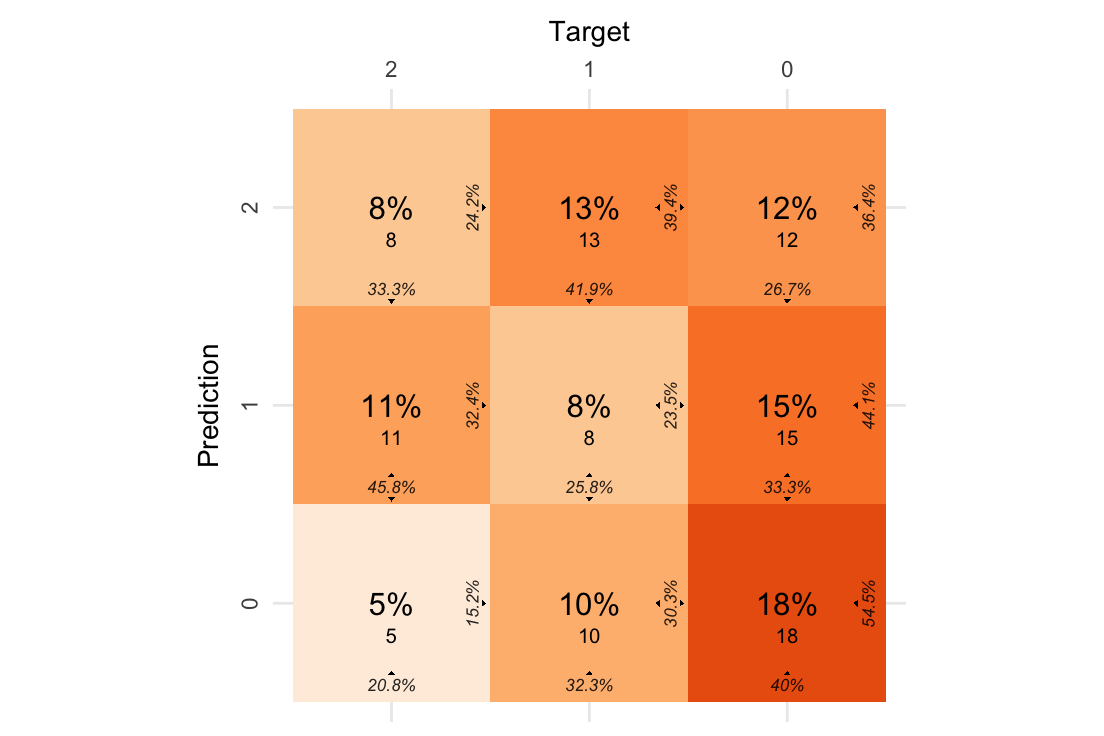plot_confusion_matrix(conf_mat$Confusion Matrix[], palette = "Greens")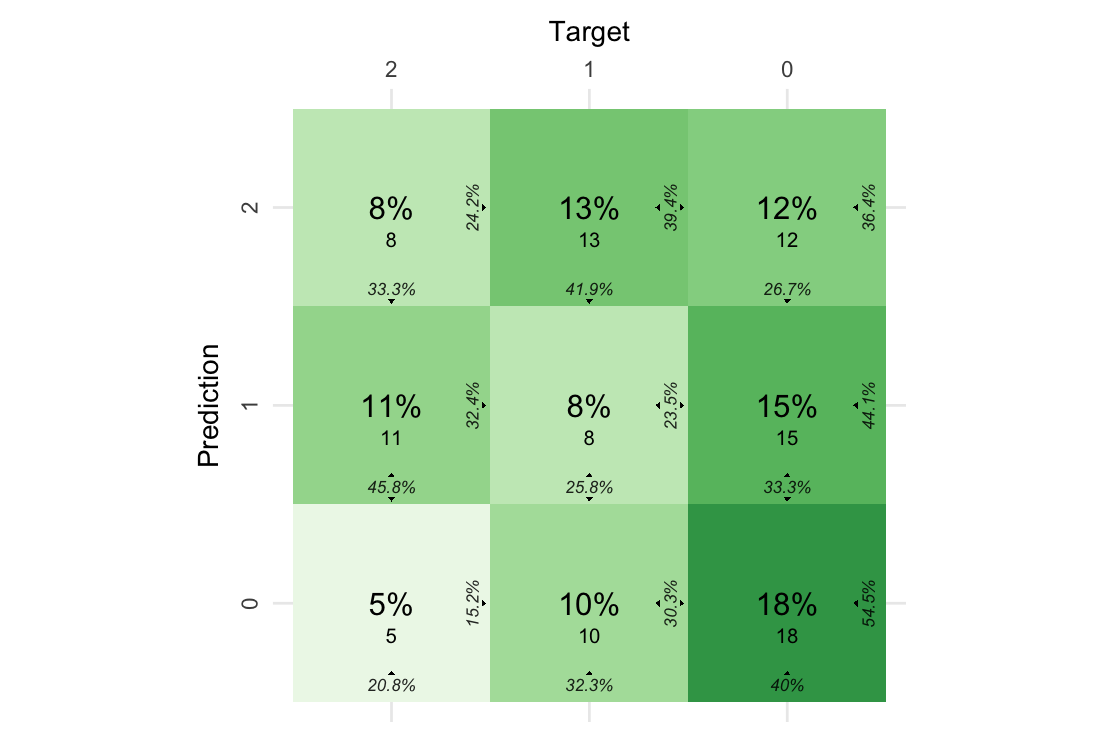When we have the sum tiles enabled, we can change the label to Total, add a border around the total count tile and change the palette responsible for the color of the sum tiles. Here we use sum_tile_settings() to quickly choose the settings we want: plot_confusion_matrix( conf_mat$Confusion Matrix[],
sums_settings = sum_tile_settings(
palette = "Oranges",
label = "Total",
tc_tile_border_color = "black"
)
)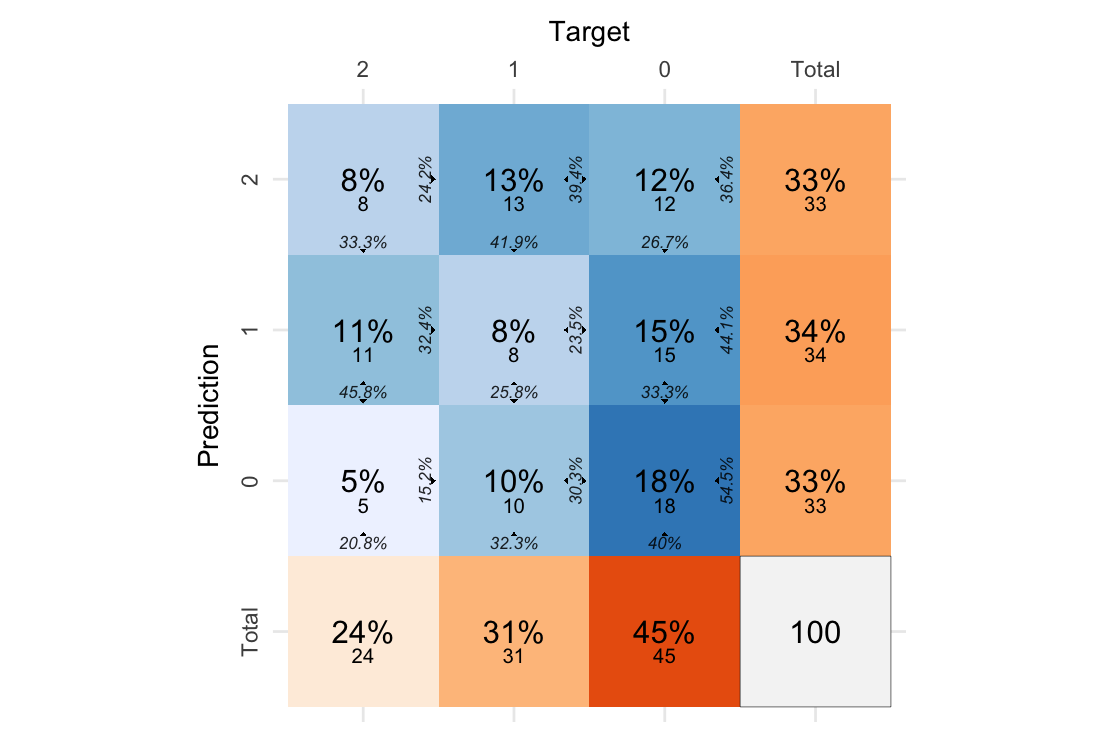Finally, let’s try tweaking the font settings for the counts. For this, we use the font() helper function.

Let’s disable all the percentages and make the counts big, red and angled 45 degrees:

plot_confusion_matrix(
conf_mat\$Confusion Matrix[],
font_counts = font(
size = 10,
angle = 45,
color = "red"
),
)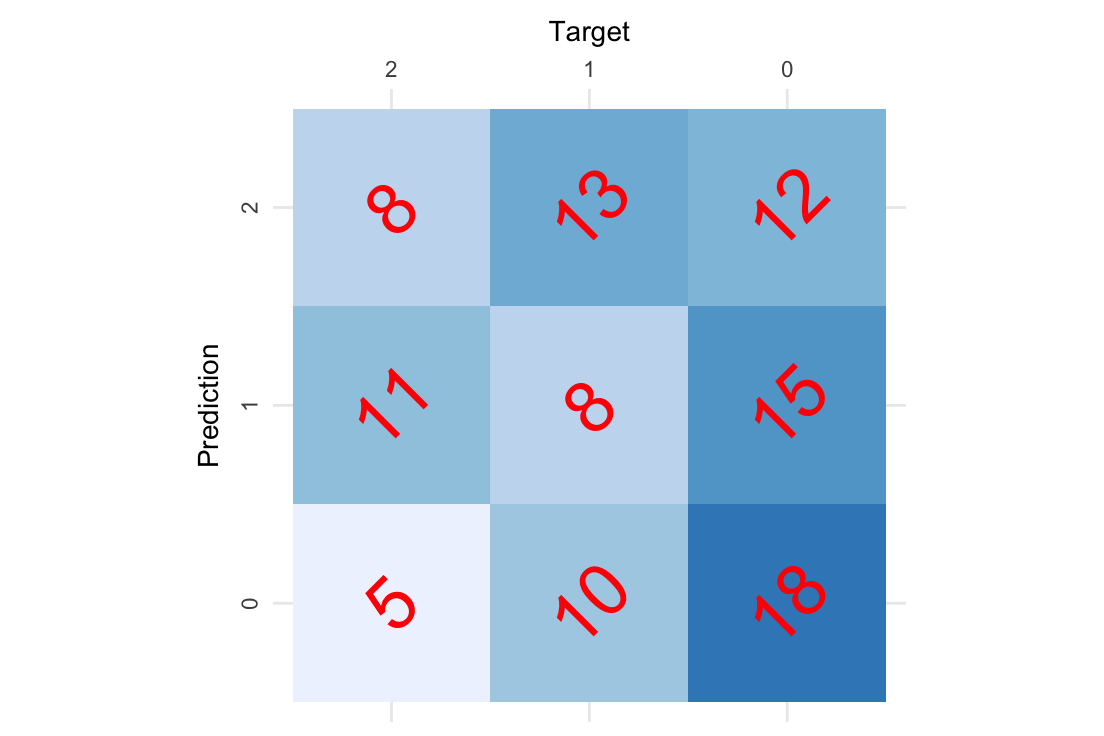Now you know how to create and plot a confusion matrix with cvms`.